#### Iteratively Reweighted Least Squares Algorithm (Fisher Scoring)

Let Y be the response variable that takes values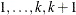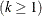. Let j index all observations and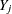be the value of response for the jth observation. Consider the multinomial variable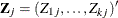such that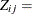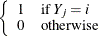and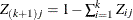. With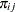denoting the probability that the jth observation has response value i, the expected value of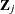is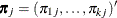, and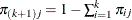. The covariance matrix ofis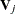, which is the covariance matrix of a multinomial random variable for one trial with parameter vector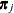. Letbe the vector of regression parameters—for example,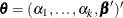for cumulative logit model. Let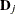be the matrix of partial derivatives ofwith respect to. The estimating equation for the regression parameters is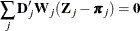where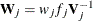, and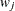and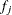are the WEIGHT and FREQ values of the jth observation.

With a starting value of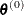, the pseudo-estimate ofis obtained iteratively as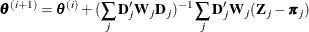where,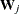, andare evaluated at the ith iteration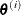. The expression after the plus sign is the step size. If the log likelihood evaluated at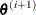is less than that evaluated at, thenis recomputed by step-halving or ridging. The iterative scheme continues until convergence is obtained—that is, untilis sufficiently close to. Then the maximum likelihood estimate ofis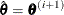.

By default, starting values are zero for the slope parameters, and starting values are the observed cumulative logits (that is, logits of the observed cumulative proportions of response) for the intercept parameters. Alternatively, the starting values can be specified with the INEST= option in the PROC SURVEYLOGISTIC statement.